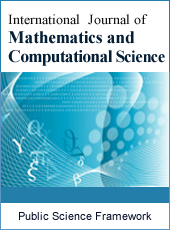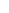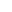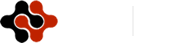International Journal of Mathematics and Computational Science
Articles Information
International Journal of Mathematics and Computational Science, Vol.4, No.3, Sep. 2018, Pub. Date: Aug. 20, 2018
Estimating Bounded Population Total Using Linear Regression in the Presence of Supporting Information
 Lamin Kabareh, Department of Mathematics and Statistics, Pan African University Institute for Basic Sciences, Technology and Innovation (PAUSTI), Juja, Kenya.
 Thomas Mageto, Department of Mathematics and Statistical Sciences, Jomo Kenyatta University of Agriculture and Technology, Nairobi, Kenya.
Estimation of finite population total using linear regression in the presence of auxiliary information is considered. Model based on linear regression model is proposed. Like the existing estimators, this estimation technique deals with QR decomposition approach based on normalized yearly population totals in order to best fit in a model within a given period of time in this study. The proposed linear regression model technique has shown to be efficient. The empirical study indicated that the linear regression model is efficient and can estimate properly when the QR decomposition approach is applied even in the presence of outliers.
Linear, Model, Estimation, Population Total, Supporting Information, QR Decomposition, Regression, Outliers
 Khan, M., Singh, R. 2015, Estimation of population mean in chain ratio-type estimator under systematic sampling. Jour. Prob. Statist. 2015, 1-5. DOI: 10.1155/2015/248374.
 Singh, H. P., Pal, S. K., Solanki, R. S., 2017, A new class of estimators of finite population mean in sample surveys. Commun. Statist. Theo. Meth., 46 (6), 2630-2637.
 Singh, H. P., Pal, S. K., 2017a, A class of exponential type estimator of a general parameter. Commun. Statist. Theo. Meth. 46 (8), 3957-3984.
 Sharma, M. K., Barar, S. S., Kaur, H., 2017, Most efficient estimators of population mean using known information of population mode of auxiliary variable. Inter. Jour. Appl. Math. Statist. 56 (5), 108-117.
 Singh, H. P., Pal, S. K., 2015, A new chain ratio-ratio-type exponential estimator using auxiliary information in sample surveys. Inter. Jour. Math. Appl. 3 (4-B), 37-46.
 Singh, H. P., Pal, S. K., 2017b, A new family of estimators of the population variance using information on population variance of auxiliary variable in sample surveys. Statist. Transit.-New Series, 17 (4), 126.
 Royall, R. M. (1970), “On Finite Population Sampling Theory Under Certain Linear Regression Models,” Biometrika, 57, 377-387.
 Hansen, M. H., Madow, W. G., and Tepping, B. J. (1983). “An Evaluation of Model-Dependent and Probability-Sampling Inference in Sample Surveys” (with discussion), Journal of the American Statistical Association, 78, 776-807.
 C. Henry Edwards, David E. Penney, 2008. Differential equations: Computing and modeling, 4th edition, 79-92.
 Hartley H, Ross A (1954). “Unbiased Ratio Estimates.” Nature, 174, 270–271.
 Al-Jararha J (2012). Unbiased Ratio Estimation for Finite Populations. LAMBERT Academic Publishing, Germany.
 Al-Jararha J, Al-Haj Ebrahem M (2012). “A Ratio Estimator Under General Sampling Design.” Austrian Journal of Statistics, 41, 105–115.
 Horvitz D, Thompson D (1952). “A Generalization of Sampling Without Replacement from a Finite Universe.” Journal of the American Statistical Association, 47, 663–685.
 Olkin I (1958). “Multivariate Ratio Estimation for the Finite Populations.” Biometrika, 45, 154–165.
 Singh D, Chaudhary F (1986). Theory and Analysis of Sample Survey Design. New Age Publication, New Delhi, India.
 Abu-Dayyeh W, Ahmad M, Ahmad R, Hassen A (2003). “Some Estimators of a Finite Population Mean Using Auxiliary Information.” Applied Mathematics and Computations, 139, 287–298.
 Kadilar C, Cingi H (2004). “Estimator of a Population Mean Using Two Auxiliary Variables in Simple Random Sampling.” International Mathematical Journal, 5, 357–367.ISSN Print: 2381-7011
ISSN Online: 2381-702X
Current Issue:
Vol. 5, Issue 2, June Submit a Manuscript Join Editorial Board Join Reviewer Team
 About This Journal All Issues Open Access Indexing Payment Information Author Guidelines Review Process Publication Ethics Editorial Board Peer Reviewers600 ATLANTIC AVE, BOSTON,
MA 02210, USA+001-6179630233
JournalsJournal ListsAuthor GuidelinesPayment InformationSubmit a ManuscriptJoin as Editorial MembersJoin as ReviewersPrivacy NoticeTerms of ConditionsHelp Center